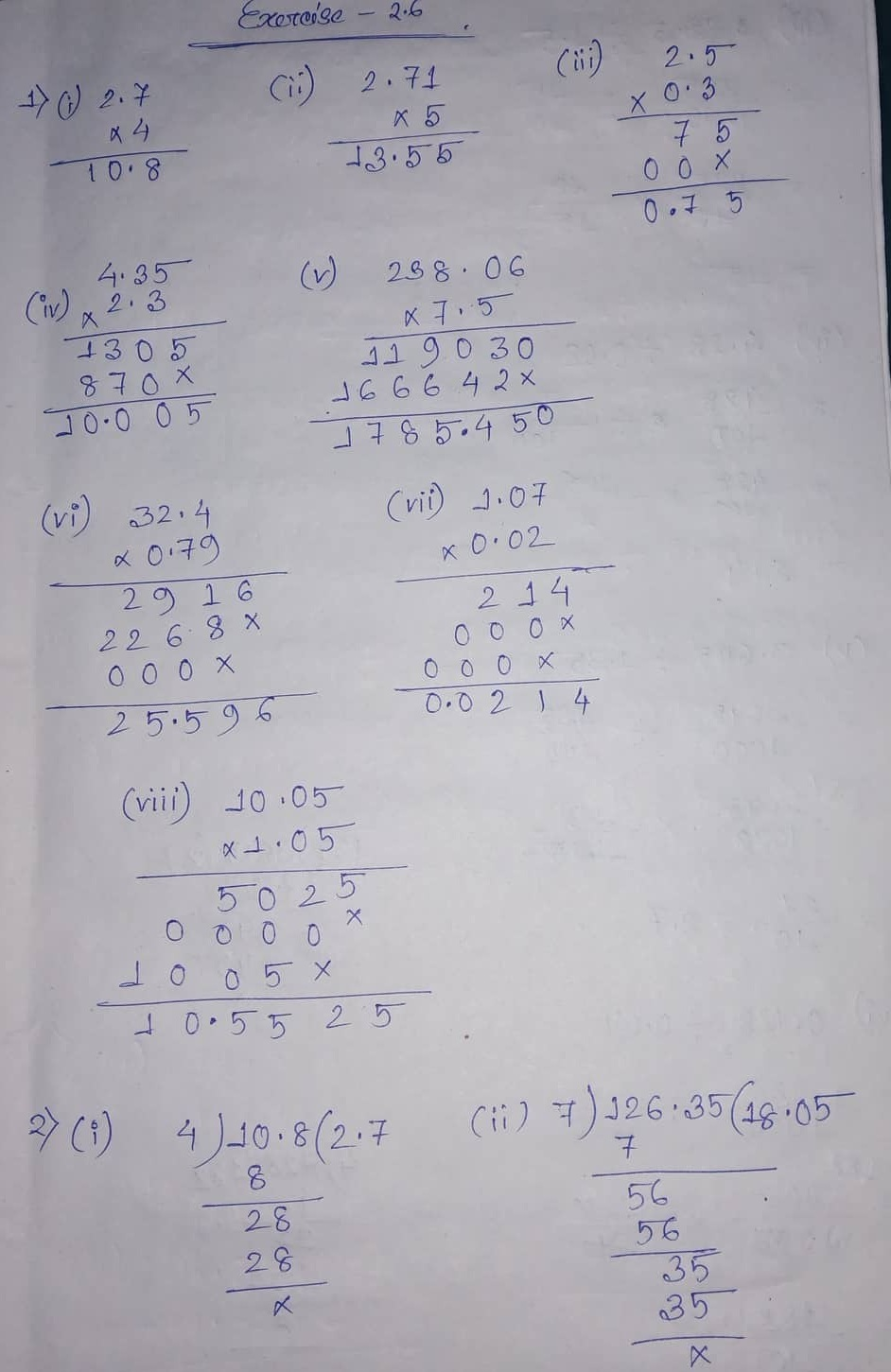# ML Aggarwal CBSE Solutions Class 7 Math Second Chapter Fractions and Decimals Exercise 2.6

### ML Aggarwal CBSE Solutions Class 7 Math 2nd Chapter Fractions and Decimals Exercise 2.6

(1) Find the following:

(i) 2.7 x 4

(ii) 2.71 x 5

(iii) 2.5 x 0.3

(iv) 2.3 x 4.35

(v) 238.06 x 7.5

(vi) 0.79 x 32.4

(vii) 1.07 x 0.02

(viii) 10.05 x 1.05

(2) Find the following:

(i) 10.8 ÷ 4

(ii) 126.35 ÷ 7

(iii) 22.5 ÷ 1.5

(iv) 4.28 ÷ 0.02

(v) 3.645 ÷ 1.35

(vi)0.728 ÷ 0.04

(v) 13.06 ÷ 0.08

(viii) 58.635 ÷ 4.5

(3) Multiply each of the following numbers by 10, 100 and 1000 (orally):

(i) 5.9

(ii) 3.76

(iii) 0.549

Solution:(4) Divide each of the following numbers by 10, 100 and 1000 (orally):

(i) 4.8

(ii) 38.53

(iii) 128.9

(5) Find the area of a rectangle whose length is 5.7 cm and breadth is 3.5 cm.

(6) The cost of one metre is Rs. 38.50. Find the cost of 3.6 m cloth.

Solution:(7) A two-wheeler covers a distance of 45.3 km in one litre of petrol. How much distance will it cover in 5.9 litres of petrol?

(8) If 1 kg of pure milk contains 0.245 kg of fat. How much fat is there in 12.8 kg of milk?

(9) If Rs. 242.46 are to be distributed among 6 children equally, find the share of each.

(10) A vehicle covers a distance of 43.2 km in 2.4 litres. How much distance will it cover in one litre of petrol?

Solution:(11) How many ice cream cones can be filled from 8.4 litres of ice cream, if one cone can be filled with 35 milliliters of ice cream?

(12) If the product of two decimal numbers is 38.745 and one of the numbers is 2.7, find the other.

(13) If 2/3 of a number is 10, then what is 1.75 times of that number?

Solution:Updated: December 5, 2019 — 2:11 pm